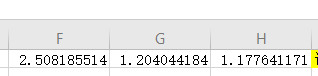# 用户运营：RFM分层实战演练(原创)R 最近一次交易的时间：R值最大，说明发生交易的时间越久，反之，发生交易的时间越近。

F 交易的频次：F越大，说明交易频繁，是平台的用户用户，反之，用户不够活跃。

M 交易的总金额：M越大，客户价值越高，是平台的重点用户。1. 先筛选数据

2. 定义RFM评估模型3. 先算出R值IF(D10<=30,5,IF(D10<=60,4,IF(D10<=90,3,IF(D10<=120,2,1))))4. 算出F值5. 算出M值6. 进一步计算IF(B6>\$F\$1,"高","低")7. 用if函数自动计算出用户层级 IF(AND(F2="高",G2="高",H2="高"),"重要价值用户", IF(AND(F2="高",G2="低",H2="高"),"重要保持用户", IF(AND(F2="低",G2="高",H2="高"),"重要发展用户", IF(AND(F2="低",G2="低",H2="高"),"重要挽留用户", IF(AND(F2="高",G2="高",H2="低"),"一般价值用户", IF(AND(F2="高",G2="低",H2="低"),"一般发展用户", IF(AND(F2="低",G2="高",H2="低"),"一般保持用户", IF(AND(F2="低",G2="低",H2="低"),"一般挽留用户"))))))))8. 做表，制定策略12-16
12-08
10-29
10-06
09-06
08-21